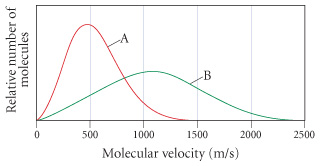# Problem: The following graph shows the distribution of molecular velocities for two different molecules (A and B) at the same temperature.Which molecule has the higher molar mass?

###### FREE Expert Solution

Recall that the molecular speed of a gas is given by:

where R = gas constant, T = temperature, and M = molar mass of the gas.

100% (158 ratings)###### Problem DetailsThe following graph shows the distribution of molecular velocities for two different molecules (A and B) at the same temperature.

Which molecule has the higher molar mass?

Frequently Asked Questions

What scientific concept do you need to know in order to solve this problem?

Our tutors have indicated that to solve this problem you will need to apply the Velocity Distributions concept. You can view video lessons to learn Velocity Distributions. Or if you need more Velocity Distributions practice, you can also practice Velocity Distributions practice problems.

What professor is this problem relevant for?

Based on our data, we think this problem is relevant for Professor Decker's class at LUC.